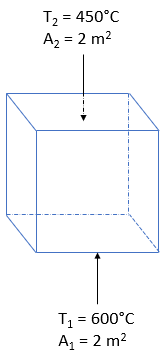## Two Black Surfaces

Consider the cubic enclosure below has an area of 2 m^2 for each face. Surface 1, which refers to the bottom black face, has a temperature of 600°C. Surface 2, which refers to the back black face, has a temperature of 450°C. If the shape factor between the two surfaces is 0.3, what is the net heat transfer rate caused by radiation? Note the Stefan-Boltzmann constant is 5.67 x 10^8W/(m^2*K^4).Hint
The net energy exchange by radiation between two black bodies that see each other is:
$$Q_{1-2}=\sigma A_1F_{1-2}(T_{1}^{4}-T_{2}^{4})$$$where $$\sigma$$ is the Stefan-Boltzmann constant [ $$\sigma = 5.67\times 10^{-8}W/(m^2\cdot K^4)$$ ], $$A$$ is the body surface area, $$F$$ is the shape factor, $$T_1$$ is the absolute temperature of the body surface, and $$T_2$$ is the absolute temperature of the surroundings. Hint 2 To convert from Celsius to Kelvin, add 273 to the temperature in Celsius. The net energy exchange by radiation between two black bodies that see each other is: $$Q_{1-2}=\sigma A_1F_{1-2}(T_{1}^{4}-T_{2}^{4})$$$
where $$\sigma$$ is the Stefan-Boltzmann constant [ $$\sigma = 5.67\times 10^{-8}W/(m^2\cdot K^4)$$ ], $$A$$ is the body surface area, $$F$$ is the shape factor, $$T_1$$ is the absolute temperature of the body surface, and $$T_2$$ is the absolute temperature of the surroundings.
$$Q_{1-2}= 5.67\times 10^{-8}\frac{W}{(m^2\cdot K^4)}(2m^2)(0.3)[(600+273K)^4-(450+273K)^4]$$$$$Q_{1-2}= 10,464.38\:W=10.46\:kW$$$
10.46 kW